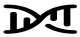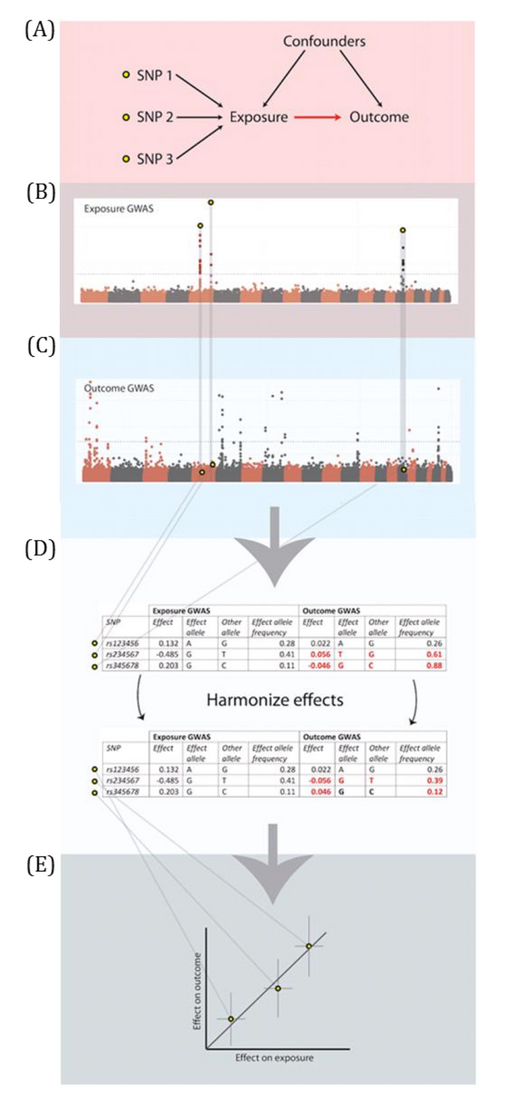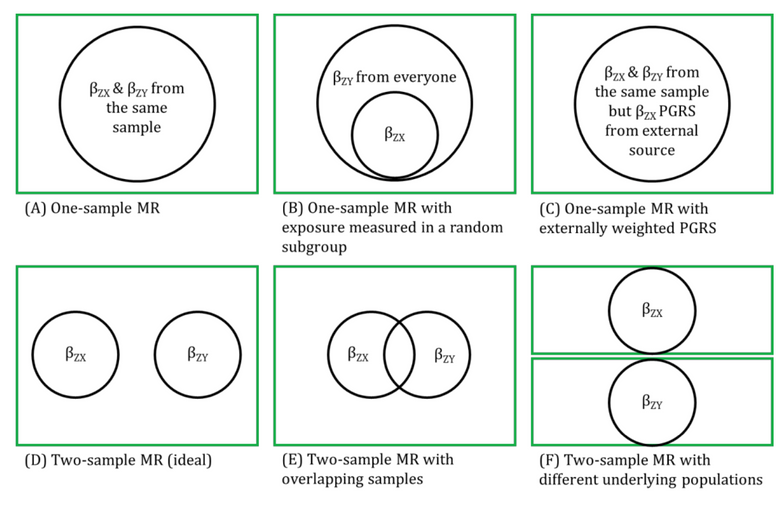Two-sample MR with summary (aggregate) data

Two-sample MR in which summary (aggregate) results are used for the genetic IV-risk factor and genetic IV-outcome associations. These are usually summary statistics from GWASs that are publicly available.

Assumptions and key sources of bias are as for two-sample MR. With aggregate data, it may be difficult to ensure the two samples are from the same underlying population. It is difficult to explore whether the genetic IVs are related to confounders of the risk factor-outcome association. It is not possible to detect non-linear effects unless there are aggregate data results for non-linear effects. Where the two samples overlap, the greater the overlap, the more the study properties become like one-sample MR.Figure 2.5 - Two-sample Mendelian randomization. Adapted from Hemani et al. (A) In two-sample MR, the associations of the instrument(s) with the exposure and outcome are derived from two independent (i.e., non-overlapping) samples. In this example, there are three SNPs acting as genetic IVs for the hypothetical exposure (i.e., SNP1, SNP2 and SNP3). (B) Manhattan plots showing the SNP-exposure estimates for each of the three SNPs are derived from a genome-wide association studies (GWAS) of the exposure variable. (C) The estimates of association between these three same SNPs and the outcome variable are then obtained from the outcome GWAS (results that are also depicted in a Manhattan plot). (D) Effects are harmonized to ensure that the ‘effect’ estimates in both the exposure and outcome GWASs correspond to the same allele (i.e., one that consistently either increases or decreases the exposure variable) for each SNP. (E) Once effects are harmonized, MR analyses can be performed. Visually, a scatter plot can be generated to represent the results, whereby the slope of the line is equivalent to the causal estimate. For example, the when using the inverse-variance weighted method, the intercept is held at zero.Figure 2.6 - Relationship of one-sample and two-sample Mendelian randomization: populations and samples. In all examples, the green box represents the same underlying population from which samples are drawn; the black circles represent the samples and the text in these summarises the source of association of genetic instrument with exposure (βZX) and association of genetic instrument with outcome (βZY). In one-sample MR (A) where βZX and βZY are estimated within the same population, there may be over-fitting of the data because the predicted (by genetic IV) values of X are then used to predict Y in the same sample. In this study type, weak instrument bias will be expected to bias towards the confounded result. In one-sample MR, it is not necessary to have exposures measured on all sample participants. For expensive exposures, these could be measured in a subsample (B). The properties and sources of bias will be broadly similar to those in (A), where exposures are measured in all participants, but the likelihood of weak instrument bias may be greater. When βZX is obtained in a one-sample MR study but with external weights (i.e., the association magnitudes taken from a GWAS to which the sample being used for the MR did not contribute), as shown in (C), over-fitting of the data is minimised. Ideally, in two-sample MR, both samples are drawn from the same underlying population but there is no overlap of participants between the two samples, as shown in (D). In this situation, data will not be over-fitted and any weak instrument bias would be expected to bias towards the null. As GWAS get larger, and with more cohorts contributing to them, the potential for overlap between samples in summary data two-sample MR becomes increasingly likely, as shown in (E). The more overlap there is between the two samples, the more effects of over-fitting and weak instrument bias become similar to those seen in one-sample MR. In figure (F), the two samples are drawn from two different underlying populations. This might occur when using MR for testing developmental origins, when βZX is estimated in pregnant women and βZY is estimated in their offspring. In that situation, it is important to consider (and ideally test) whether the βZX association in pregnancy is the same as in non-pregnant females and males (i.e., as in the offspring sample). Similarly, when using aggregate data in two-sample MR and when the outcome of interest can only occur in one sex (e.g., cervical or prostate cancer), ideally one would want aggregate βZX estimates to be sex-specific. If that is not possible, then drawing on other external evidence to consider the extent to which βZX is likely to be similar in females and males is important.